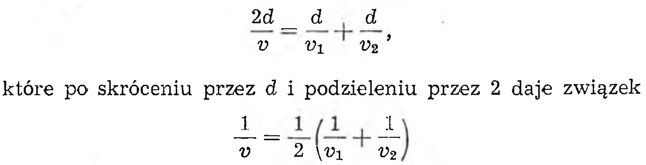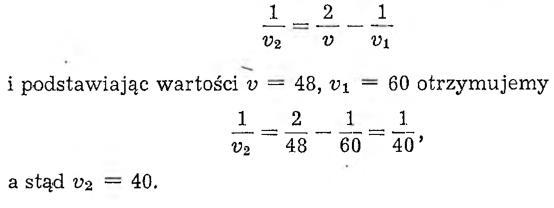# Regular drivingThe car driver took part in a regular driving competition. According to the regulations, a certain section of the road should be driven at an average speed 48 km per hour. Meanwhile, he was racing halfway down the road 60 km per hour. To what number must the driving speed decrease on the other half of the road, so that the average speed drops to 48 km per hour?

If you think, that the other half of the way the motorist would have to slide at speed 36 km per hour, then you are wrong,
although (60+36)/2 = 48.

Suppose, that the whole way was 120 km. The motorist has qualifications for the competition 120 : 48 = 2½ hours - not less, no more. So since the first half of the way, or 60 km, drove within an hour, this left him 1½ hours for the second half of the journey, so he had to go fast 60 : 1½ = 40 km per hour.

Let us consider the matter more generally. Suppose, that the length of the entire road was 2d and that the motorist traveled the first half of the road at a speed of v1, and the other half at v2. Let's calculate, what was the average speed v on the entire road.
The motorist spent the first half of the journey time d / v1 , and the other half of d / v2, so it used up d / v1 all the way + d/v2.

On the other hand, for the road 2d at driving speed v, time is needed 2d / v.

We get the equationIt turns out, that the reciprocal of velocity v is the arithmetic mean between the reciprocal of velocities v1 and v2. We speak in that case, that the speed v is the harmonic mean between the speeds v1 and v2.

In our task we have the data v = 48 i v1 = 60, and you need to determine v2.

From the above equation we calculate